PSABM5WD - Practice---Acc7.5 Lesson 9 Slopes and Equations for All Kinds of Lines (7.G.A, 8.EE.B, 8.EE.B.6)
Part A)

Calculate the slope of the line.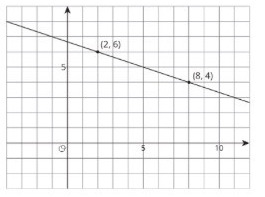Type your answer below as a number (example: 5, 3.1, 4 1/2, or 3/2):
Part B)

Calculate the slope of the line.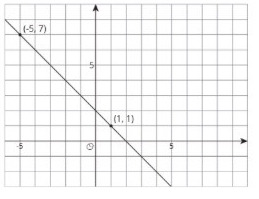Type your answer below as a number (example: 5, 3.1, 4 1/2, or 3/2):
Part C)

Calculate the slope of the line.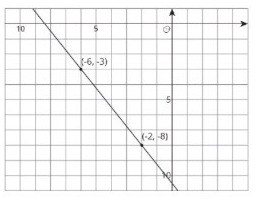Type your answer below as a number (example: 5, 3.1, 4 1/2, or 3/2):
Part A)

Match the pair of points with the slope of the line that joins them.

(9,10) and (7,2)

Select one:
Part B)

Match the pair of points with the slope of the line that joins them.

(-8,-11) and (-1,-5)

Select one:
Part C)

Match the pair of points with the slope of the line that joins them.

(5,-6) and (2,3)

Select one:
Part D)

Match the pair of points with the slope of the line that joins them.

(6,3) and (5,-1)

Select one:
Part E)

Match the pair of points with the slope of the line that joins them.

(4,7) and (6,2)

Select one:
Part A)

Draw a line with the given slope through the given point. What other point lies on that line?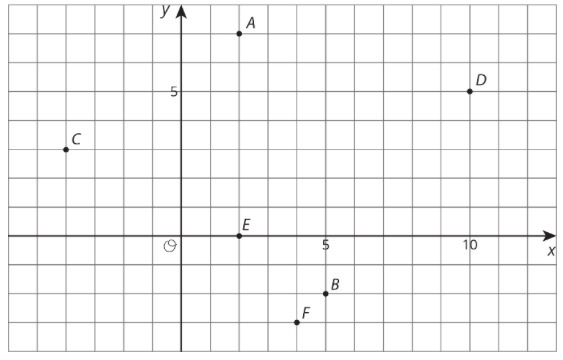Point A, slope = -3

Part B)

Point A, slope = -1/4

Part C)

Point C, slope = -1/2

Part D)

Point E, slope = -2/3

Part A)

Suppose you wanted to graph the equation -4− 1.

Describe the steps you would take to draw the graph.

Part B)

How would you check that the graph you drew is correct?

Write an equation for each line.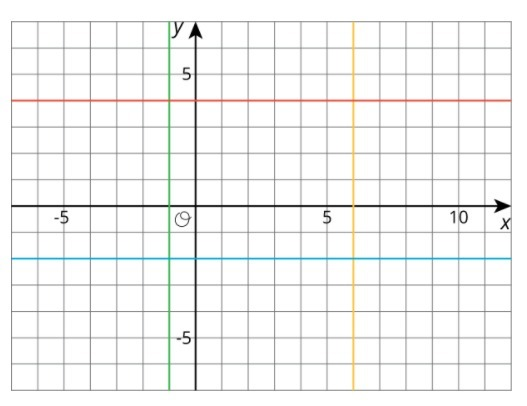Part A)

A publisher wants to figure out how thick their new book will be. The book has a front cover and a back cover, each of which have a thickness ofof an inch. They have a choice of which type of paper to print the book on.

Bond paper has a thickness ofinch per one hundred pages. Write an equation for the width of the book, y, if it has x hundred pages, printed on bond paper.

Complete the equation below

y = _______________

Part B)

Ledger paper has a thickness ofinch per one hundred pages. Write an equation for the width of the book, y, if it has x hundred pages, printed on ledger paper.

Complete the equation below

y = _______________

Part C)

If they instead chose front and back covers of thicknessof an inch, how would this change the equation to part A?

From Part A:

Bond paper has a thickness ofinch per one hundred pages. Write an equation for the width of the book, y, if it has x hundred pages, printed on bond paper.

Complete the equation below

y = _______________

Part D)

If they instead chose front and back covers of thicknessof an inch, how would this change the equation to part B?

From part B:

Ledger paper has a thickness ofinch per one hundred pages. Write an equation for the width of the book, y, if it has x hundred pages, printed on ledger paper.

Complete the equation below

y = _______________